# Mechanics Basics Part 2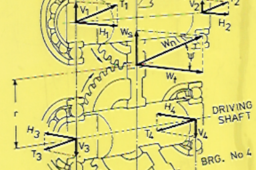In this tutorial we will look at the concept of STRESS in an engineering concept. Stress is the mechanics relationship that defines the load we place on a part, and the ability of the material it is made from, to resist this load that has been applied to it. The actual strength of a material is a physical property of the material and as the mechanical parts that we design come in all shapes and sizes, we require value, that relates our load back to the physical properties of the material we want to make our part from

1. ### Step 1:

Stress is defined as :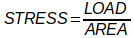This is an obvious choice for the relationship we use to define the ability of the material we are using to make our part from and the load we have applied to this part. As in the modern world there is a well thought out system of units, the S.I, unit system, a system of engineering – scientific units that has actually been designed instead of just thrown together, unlike the old British Imperial Units, were we have units such as B.T.U’s, horsepower, pounds, tons, long tons, short tons, pounds, stones, all manner of units with no simple connection to each other. Even the British who invented these units have had the good sense to replace them with the S.I. unit system.

As with all of the S.I. units everything is based on kilograms, meters and seconds. In Mechanics Basics Part 1 we derived the unit we used for a force ( load in our equation) and as the unit used for distance is the meter we have the following :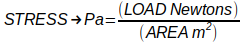The unit we use for stress has been given the name : Pascal after Blaise Pascal the French mathematician, physicist, inventor and writer. The Pascal, Pa standard abbreviated form, is also used to measure pressure as stress and pressure are based on the same things a load acting over an area.. I have seen a question asked on a GrabCad forum where the author of the question has been confused by this similar use of the unit Pa. When we are dealing with pressure we sometimes use the unit – bar. A bar is a unit used when dealing with atmospheric pressure, with 1 bar being the approximate pressure of our earth’s atmosphere. When dealing with pressure vessels we often use this bar unit. Often when specifying a pressure vessel we may state it needs to withstand a pressure of 100 bar or a pressure 100 times more than atmospheric pressure. Applying the unit bar to engineering problems involving pressure is acceptable, but using the unit, bar when working out stress problems is not good practice. 1 Pa is defined as : the force of 1 N acting on an area of 1 m2 , so a Pa is a very small unit so when dealing with stress values in the real engineering environment it is common to deal with stress in terms of MPa, or :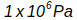The S.I. system, based on the meter as the unit for length so 1MPa is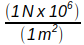but as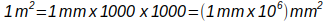his is convenient for us as from this we can work out that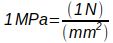this means that by working out our load supporting area in mm2 our calculated stress will be in MPa

2. ### Step 2:

We will look at applying a simple load to a body. We will take a 16mm dia mild steel rod, 300mm long, fixed at one end, and along the 300mm length, we will apply a tensile load of 2.0 KN as per Diagram 1.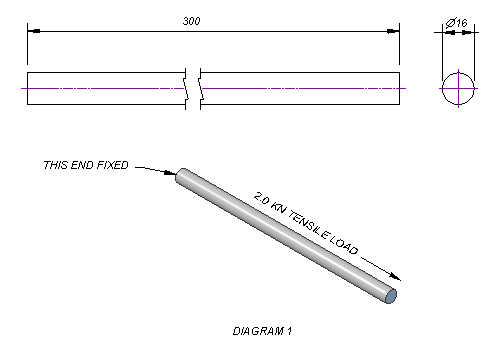This load will apply a STRESS to this 16mm dia mild steel rod. The ability to support this loading is a property of the material that the rod has been made from. We as engineers need to design our part to be strong enough to resist the stress this 2.0 KN load places on this part. We calculate the stress due to this tensile load as follows :->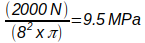As the mild steel we intend to make this part from has a yield strength of 250This part will only be lightly stressed if, in service all it has to deal with is a load of 2 KN, tension, applied along its length as in Diagram 1. Using this standard formula it id a quick and simple task to check the stress in this part.

It is IMPORTANT that the stress in this part is only valid for a tensile or pulling force. If the force applied is reversed, and becomes a compressive force, the stress calculated in this way is no longer valid. When the direction of our load is reversed from a tensile to a compression force we now have a different situation. Our 16mm dia rod, having a length much larger than its diameter lacks rigidity when subjected to a compression force. It is possible to pull a load along with a chain or a rope but ropes and chains can’t be used to push a load.

For relatively slender parts, where the length is much greater than the width, and made of ductile materials, like mild steel, that fail under to compressive loads, it is mainly due to buckling. Parts made from ductile materials that have a relatively short length compared to width will fail due to the direct compressive stress. Brittle materials such as cast iron tend to fail suddenly due to the shear stress induced by the compression load. Analyzing the stress in struts and columns is a more advanced topic than the simple concepts we are looking at in this tutorial.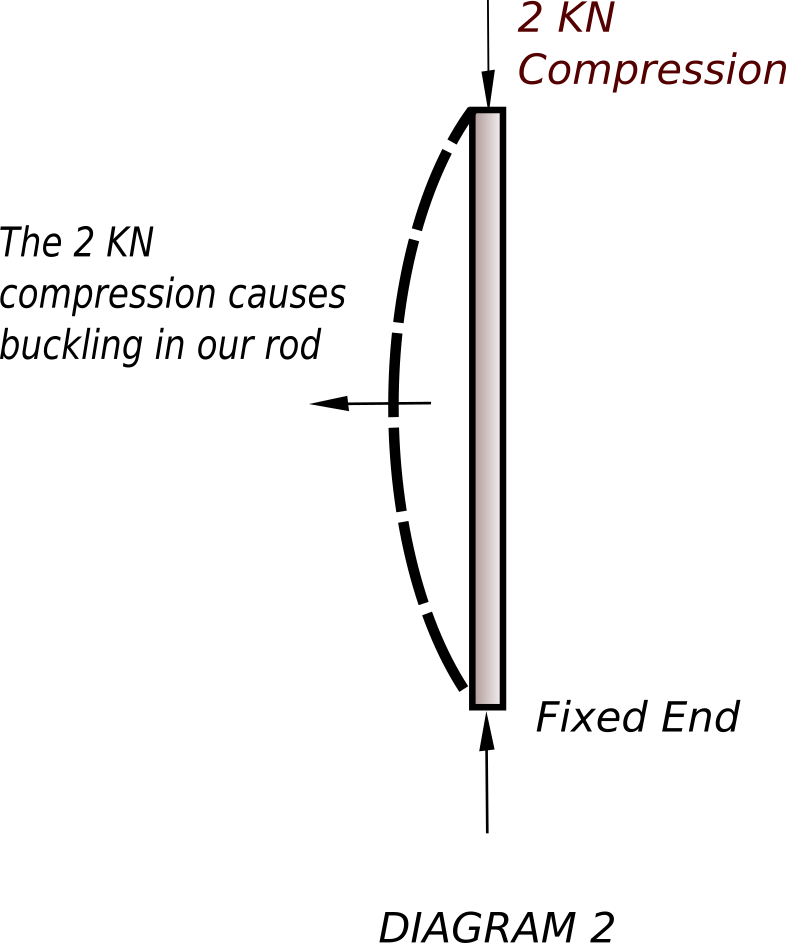3. ### Step 3:

Looking at mild steel as a material, we will now take a quick look the basic properties of mild steel as an engineering material. When we select a particular steel to make our required part we make our choice based on the mechanical properties of our chosen material. One of the basic methods used to determine the properties of a particular steel is to subject a sample to a standard tensile test.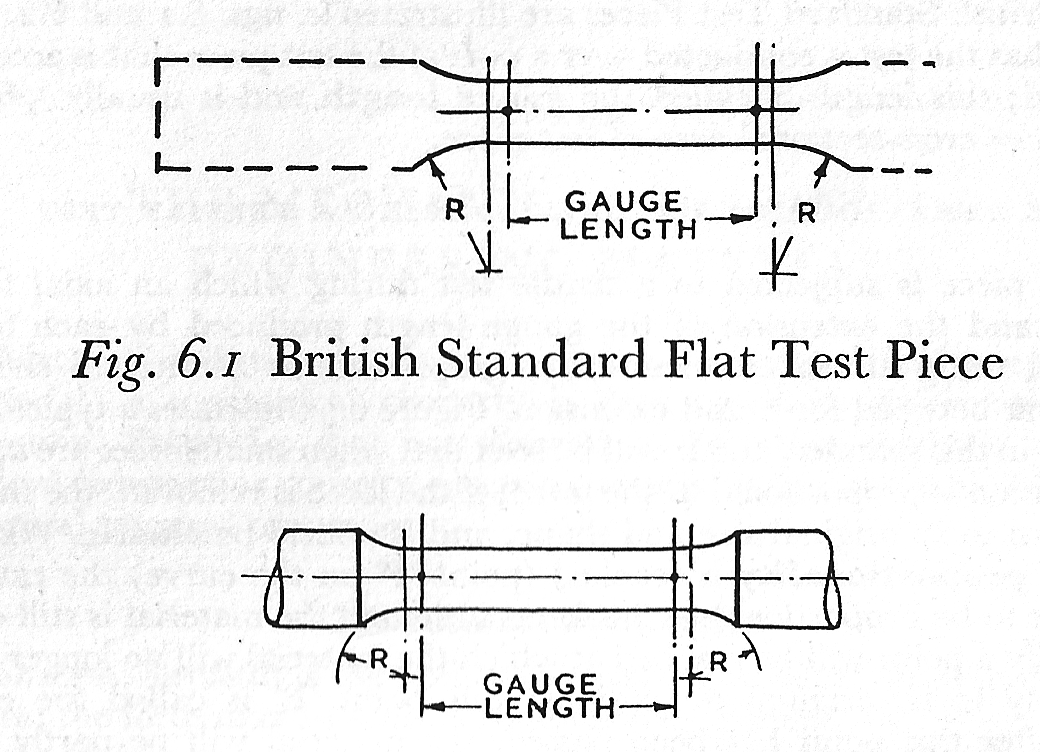Diagram 3 shows a sketch of a test piece that will be used to determine the properties of a steel sample. This sample will be placed in a tensile testing machine and the applied load increased until the material fails. As the load is applied accurate measurements are taken of the gauge length marked on the sample.